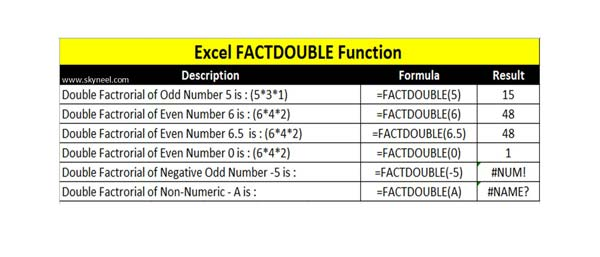# Excel FactDouble Function – Return Double Factorial of Number

Excel FactDouble Function is located in Math & Trig category. Factdouble allows you to returns double factorial of given positive number.

Excel FactDouble function is used to returns the double factorial of given positive number. The FactDouble function in Excel is located in the Math & Trig category. The double factorial of any positive number is equal to 6*4*2…. number, while for any negative number is equal to 5*3*1.. If you need to quickly calculate double factorial of any large even or odd number then you can use Excel FactDouble function in active worksheet. In this article we have to describe the formula and how to use Excel FactDouble function.

The Microsoft Excel FactDouble function allows you to quickly returns the double factorial of any positive even or odd number. FactDouble is an important built-in function which is located in the Math & Trig category. This function can be used just like a worksheet (WS) function. You can use FactDouble function to get the double factorial either directly fill the number or use cell address in active worksheet. In our previous article we have already discuss about the Excel Fact function which returns factorial of given number.

Syntax of Excel FactDouble Function

FACTDOUBLE(number)

The FACTDOUBLE function has only number arguments:

Number: Required. You have to give any no-negative number for which you want the double factorial. If given number is not an integer then, decimal portion has been truncated.

If give number is even then n=n(n-2)(n-4)…(4)(2) and given number is odd then n=n(n-2)(n-4)…(3)(1)

If you try to calculate double factorial of any negative number then you will get #NUM! error message in active cell. Just like this you can also get #NAME? error message when you try to calculate double factorial of any non-numeric value in active cell. So, you must always remember FactDouble function return the double factorial only of any positive number. If you have any number with decimal value then decimal places are removed and double factorial calculated only on non-decimal value.

## How to use Excel FactDouble Function

If you want to use Excel FactDouble function in your active worksheet then you have to check this.If you want to calculate double factorial of any positive odd number like 5 then you have to type the given function in any cell: =FACTDOUBLE(5) and press enter you will get 15 as result.

If you want to calculate double factorial of any positive even number like 6 then you have to type the given function in any cell: =FACTDOUBLE(6) and press enter you will get 48 as result.

Most of the person want to know the double factorial of 0 number then you have to use given function: =FACTDOUBLE(0) and press enter you will get 1 as a result.

If you try to calculate double factorial of any negative number or non-numeric value then you will get an error message in active cell.

=FACTDOUBLE(-5) returns #NUM! error value.
=FACTDOUBLE (A) returns #NAME? error value.

Always remember if you want to become an Excel expert then you must have better knowledge of Excel functions. I hope after reading this guide you can easily use Excel FactDouble function. If you have any query or suggestion then send us via comment box.